## Tuesday, 29 September 2009

### W' oops fram alpha?

I like Wolfram|Alpha. I use it in my classroom and even recommend it to my students as a great way to play around with mathematical ideas at home... but sometimes I get surprised at the strange things it won't do.

For instance, this morning in class.

We are working with inverse relations with my kids; trying to show that every mapping has an inverse mapping, but that doesn't mean either one of them is a function... and I showed them a complicated implicit function. I started withx^2 + 3xy + 2xy^2 = 7 just picked something off the top of my head...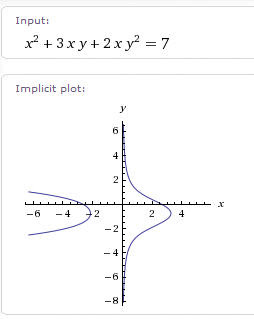It was interesting, then we talked about what it would look like if we flipped it around by interchanging the x's and y's.

y^2+3yx+2yx^2=7 but???I couldn't imagine what was amiss, but retyped it as y^2 + 3xy + 3x^2 y=7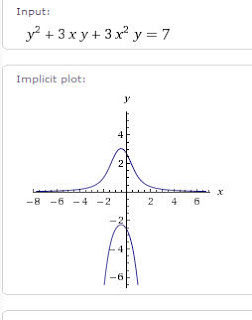Curious, I experimented...was it the order yx verses xy that confused it? tried a rectangular hyperbola

xy=1 no problem.... yx=1 this does not compute???

Ok, so what happens if I type in x=y^2... I get the typical parabola orientation you would expect with y=x^2, centered at the origin and opening up along the y? axis. Neither axis was labeled in this image, but the graph is labeled "y from -1 to 1"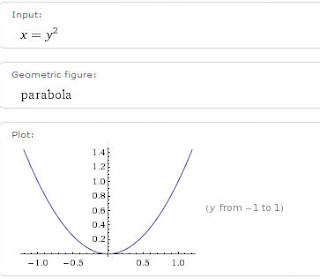y^2 = x shows the right opening parabola you would expect, with both axes labeled.

One of the many idiosyncrasies of Wolfram alpha.

## Monday, 28 September 2009

### Mathematical Induction, A Brief History of the Term

I have had an interest in the history and etymology of mathematical terms for many years, as witnessed by my MathWords web page. Recently I came across a couple of old journal articles, (1915-1918) related to the history of mathematical induction, and to the term itself. Most of this comes from an article by Cajori, and the very early dates above. Certainly those who know more about the current status of the usage could help by sharing their information.

The logical and scientific process called induction dates back as far as Cicero's translation of Aristotle. Cicero used the latin term "inductio", for the Greek "epagoge", which translates as "leading to."
Induction has always existed in mathematics, but the formal concept of mathematical induction did not appear until it was developed by Maurolycus in 1575 to prove that the sum of the first n odd numbers is n2. While the roots of formal mathematical induction are nested in works from Fermat all the way back, one might say, to Euclid's proof of the infinity of the primes, the work of Maurolycus was unique in the formal use of attaching one term to the next in a general way.

The method of Maurolycus was repeated and extended in the works of Pascal to be a much more clear illustration of the present method but none of them used a particular name for their logical process. Then in his Arithmetica infinitorum in 1656 Wallis decided to name the term. On page 15 he creates the term "per modum inductionis" to prove that the limit of the ratio of the sum of the first n squares to n3 + n2 was 1/3. His inductive method followed very much the unnamed method of Maurolycus.

Later Bernoulli gives an improvement to Wallis' method by showing the argument from n to n+1 as a general proof; this was the real foundation of modern mathematical induction. Bernoulli gives no specific name to his process, but uses his method as an improvement on the "incomplete induction" earlier used.

For the next 150 years, mathematicians used induction in both senses, to refer to the process of observing a relationship from a pattern , and in the method of Bernoulli to prove such an induced relationship by arguing from n to n+1. Then early in the 19th century, George Peacock uses the term "demonstrative induction" in his 1830 Treatise on Alebra. Then several years later, Augustus De Morgan proposes the name "successive induction" but then at the end of the article he talks about the method as "mathematical induction."

Isaac Todhunter used both names in his chapter on the method, but he used only Mathematical Induction in the chapter heading. Several popular textbook authors, Jevons and Ficklin, for example, used both terms. But among several others, Chrystal, Hall and Knight, used only the term mathematical induction. The same name seems to have been common in the early part of the 20th century in America and Europe, with Germany seemingly clinging to a single term for both "complete" and "incomplete" induction. Cajori, in 1918, says the Germans most commonly use the term, "vollstandige Induktion". I do not know if there is currently a more appropriate notation for the true mathematical induction of Bernoulli in Germany. If a reader is familiar with the current situation in German mathematics, please update me.

## Sunday, 27 September 2009

### Student Confusion about Order of OperationsIn America they say "PEMDAS" and the British often use "BODMAS" and some decry any mnemonics at all, but everyone teaches essentially the same rules of evaluation.

Consider the poor Algebra I or II student, who has seen and heard the following in his first few weeks of school:

"Multiplication and Division are handled as equals, from left to right."

"When we say 6a we mean 6 times a, where a just represents any number that we might choose to be use in place of it"

And then later we write on the board 6a2 / 3a = ?
and so the student says, "I get it, that means 6 times a2 then I divide by 3 and multiply by a. It must be 3a3."
Then, wanting to follow instructions, he types the expression into his Ti-84 + silver edition calculator after storing a value for x, and sure enough, he gets the value of 3x 3.

But of course, you explain, that's wrong. when we divide like this we mean that the 3a is intended to be a monomial term, taken as a single unit.
Your student is compliant, so he/she just nods and murmurs "Ok" and as you walk back to the front of the room, turns to their neighbor and says "You understand that?" and gets a shrug and a side to side head-shake... all just part of the mystery of mathematics.

If you realize what is confusing them, you may become very conscientious about writing problems with the fractions written out with horizontal fraction bars $\frac{6x^{2}}{2x}$ and talk about "implied parentheses". Unfortunatly many of your students have only heard the term "implied" used in prejudicial situations;"He implied I had stolen it!" or similar, and really have no idea what you mean. So maybe you get REALLY conscientious and write every fractional expression using actual parentheses $\frac{(6x^{2})}{(2x)}$.... and then that night on the homework, the book does NOT use them.

The repercussions of this order of operations confusion leads to students unsure of whether to write the linear term in an equation as $\frac{5x}{2}$, or 5$\frac{x}{2}$ or $\frac{5}{2}$ x.. but sometimes that even leads to $\frac{5}{2x}$ .

## Friday, 25 September 2009

I've mentioned before my admiration for micro-credit (see the big Kiva link in my sidebar... go on, kick in $25 and make the world a little better place). Here is a wonderful talk from Jacqueline Novogratz(say that three times as fast as you can!) about what she has termed as patient capitalism.. A middle ground between profit at all risk capitalism and charity that too often forces the poor into a worse position than they started from. It's not enough to talk about world peace, you gotta make it happen... Hope you enjoy it ## Wednesday, 23 September 2009 ### An Interesting Observation, and a ProblemI came across a couple of neat things reading old journals sent to me by Dave Renfro... here are two that seem worth sharing: In the Mathematical Spectrum in 1984, Malcolm smithers of the Open University shared a discovery... "I found the following interesting number on my Oric 1 48K" (The Oric1 (16k) was released in 1982 and was the first color computer to sale for under 100 GB Pounds..I still remember working with my son as we both learned computing on a Tandy color computer... alas he has left me in the dust computer wise) "3435 =$3^{3} + 4^{4} +3^{3} + 5^{5}$" which left me wondering, is there any number between this number and 1=$1^{1}$that replicates this kind of behavior.. none came quickly to mind, and I was too busy to do a computer search. Here is an interesting problem from the American Mathematical Monthly from May, 1930: A piece of pie is cut from a pie with a radius r. The cut is a sector with a central angle of$\theta$. Assuming that the angle$\theta$is < 180o. What is the radius of the smallest plate that will hold the cut piece of pie? Have fun. For the calculus student, is the function differentiable over this domain? Enjoy ## Tuesday, 22 September 2009 ### Powers of three.... Continue the Pattern 1 --------------------------- =$3^{0}$1 + 2------------------------ =$3^{1}$2 + 3 + 4 ----------------- =$3^{2}$2 + 3 + 4 + 5 + 6 + 7 ------- =$3^{3}$5 + 6 + 7 + .... + 13 ------- =$3^{4}$5 + 6 + 7 + .... + 22 ------- =$3^{5}\sum_{n=14}^{40}{n} =3^{6}\sum_{n=14}^{67}{n} =3^{7}\sum_{n=41}^{121}{n} =3^{8}$and then???? Can you write a general expression? From the Mathematical Spectrum, 1983, submitted by L. B. Dutta of Keshabpur, Bangladesh ## Monday, 21 September 2009 ### The Hypotenuse of a Right Triangle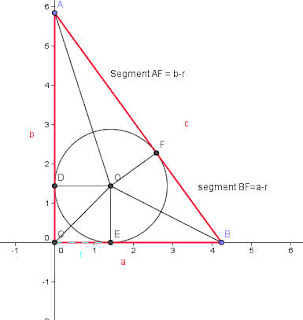In my last blog I challenged the student to find the length of the diameter of a circle inscribed in a right triangle in terms of the three sides, a, b, and c. Students often let the "Right Angle" divert them into trying to find something to do with the Pythagorean Theorem, but as I shall show, that is really just putting the horse before the cart. In this case, all they need to see is that the two segments from each vertex are both tangent to the circle, and apply a pretty well known property of tangents from a point. Using this idea, it seems clear from the image above that Segments AD and AF are congruent, and by looking at the vertical leg of the triangle, we see that both of these are of length b-r. In the same way the segments BE and BF are also congruent, and they both have a length of a-r... but AF + BF = c, so c=a-r+b-r, or c= a+b-2r... Now just add 2r and subtract c from both sides and we get 2r (the diameter of the circle) = a+b-c. Of course you could have started out with a look at the area. Since the triangle is made up of three smaller triangles, COB, BOA, and AOC. Each of these areas is 1/2 the product of a side of the triangle and the radius of the circle. So A= 1/2 ar+ 1/2 br+ 1/2 cr which simplifies to A= 1/2 (a+b+c)r ... now expressing the area A as 1/2 ab, we have ab=(a+b+c)r and dividing by the perimeter, we get$\frac{ab}{a+b+c} =r$. We need only to double that to get the diameter, D=$\frac{2ab}{a+b+c}$. If we put those together, we get a proof of the Pythagorean theorem... Since the Diameter is equal to both a+b-c and$\frac{2ab}{a+b+c}$then the two expressions must be equal to each other... so ..... We begin with$\frac{2ab}{a+b+c} =a+b-c$we multiply through both sides by (a+b+c) to get 2ab= (a+b-c)(a+b+c). Think of this last as [(a+b)+c][(a+b)-c] and so we get 2ab= (a+b)2 -c2. Now we square the binomial (a+b) to get 2ab= a2+ 2ab+ b2 - c2. Subtract 2ab from both sides, and we get a2+b2-c2=0... or more simply a2+b2=c2 I love it when stuff like that happens... I should point out for those who couldn't find it, that the difference in the two equilateral triangles formed in the Napoleon Theorem, will always equal the area of the original triangle. ## Saturday, 19 September 2009 ### A Trio of Triangle Problems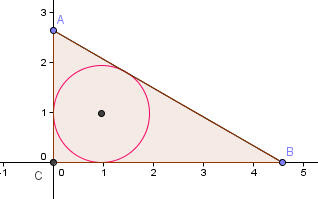In the January, 1929, issue of the American Mathematical Monthly there appears a problem submitted by J. Rosenbaum of Milford Connecticut. The problem begins, "It is well known that the radius of the inscribed circle of a right triangle is equal to half the difference between the sums of the legs and the hypotenuse." I ignore for now the problem posted, but suggest that the theorem suggested may be less well known now than it might have been in the past. But the challenge... prove it is true. I won't spoil it now, and will wait for a reader to supply a good proof... and if none appears, I will post a proof here in a day or two.A second "well known" theorem in the same article included a theorem I call a "Pythagorean look-alike". In a right tetrahedron [a triangular pyramid in which three right angles meet at a single vertex... imagine one point, A, being at the origin of the three-dimensional coordinate grid and points A, B, and C being on the x, y, and z axes at (b,0,0,) (0,c,0) and (0,0,d) ] Then the sum of the squares of the areas of the three triangles meeting at the triple right vertex is equal to the square of the area of the triangle BCD. Once more the challenge is to derive the given relationship.A popular theorem to introduce to students is Napoleon's Theorem: if equilateral triangles are constructed on the three legs of any triangle, the centers of the three new triangles will also form an equilateral triangle. You can actually do that two ways, by constructing them on the exterior of the triangle (shown above), or by constructing them on the interior sides of each leg. So here is the problem, what is the difference in the areas of the equilateral triangles on the exterior and interior. ## Monday, 14 September 2009 ### A Graphic Solution to the 25 Stones ProblemI recently wrote a blog about the problem below from the Gazette from the Australian Mathematical Society. I started out not knowing how to solve it, figured out how to solve it, but had to have Joshua Zucker give me the proof... a good learning experience, but it is such a nice problem that I wanted to see if I could explain it in terms that would make it clear to my students, so here is the problem, and a hopefully understandable solution: Piles of stones There are 25 stones sitting in a pile next to a blackboard. You are allowed to take a pile and divide it into two smaller piles of size a and b, but then you must write the number a×b on the blackboard. You continue to do this until you are left with 25 piles, each with one stone. What is the maximum possible sum of the numbers written on the blackboard? I figured out pretty quickly by experimentation that it didn't matter how you divide the piles, you ended up with the same total on the board, 300. One of the classic ways of exploring a problem like this is to make it smaller, so I reduced the pile to two, then three, etc and realized that each time the solution was equal to the number of ways of choosing two things from a collection of n things;$\dbinom{n}{2}$n ____2_______3________4_______5______6_____________________25____________n sum ___1______3_________6______10_____15____________________300__________(n)(n+1_/2 But why??? Enter Joshua with an explanation. He illustrated the solution with an analogy to a well known type of problem, the handshake problem. I will try to use basic Graph Theory... To begin with, we reduce the problem to eight stones, a more manageable number to graph... the extension to any other number should be reasonably easy... Visualize each stone as a node of a graph and lines connecting each pair, as in the complete graph of K8 shown at the top. If we count the number of line segments we will get 8 choose two,$\dbinom{8}{2}$= 28 segments, as we would expect, since each segment is formed by selecting two of the eight points to be endpoints in every possible way. Now what happens if we divide the eight points into two groups. We illustrate that by drawing a line to divide the eight points into two sets. In this example we have chosen to divide them into sets of five and three as shown here.If we count, we notice that 5x3=15 segments are cut by the line, that is, the number of connections between the separated groups is equal to the product of the size of the two groups. Eliminating these 15 segments, we get two separate groups, each of which can be subdivided again.If we now subdivide any of the other groups, we will cut another set of line segments. In this example we cut the five group.This cut will eliminate another 6 of the segments, and now there are three groups. At each stage as we reduce the groups toward one in each set, we eliminate one or more of the 28 line segments, until at the end we have eight isolated points, and all 28 line segments have been cut. Thanks Joshua, a great approach. ## Friday, 11 September 2009 ### Problems From the Land Down UnderLooking through the Gazette of the Australian Mathematical Society , and found their puzzle corner... really nice problems. I think I have this one, but I didn't prove it.... Digital deduction The numbers 2^2009 and 5^2009 are written on a piece of paper in decimal notation. How many digits are on this piece of paper? And this one has me puzzled (which is why they call them puzzles, I guess).. Piles of stones There are 25 stones sitting in a pile next to a blackboard. You are allowed to take a pile and divide it into two smaller piles of size a and b, but then you must write the number a×b on the blackboard. You continue to do this until you are left with 25 piles, each with one stone. What is the maximum possible sum of the numbers written on the blackboard? Anyone know how to a) prove the first, or b) solve the second... Do let me know....mostly down to chewing my pencil tips now.... Spoiler (I think) x x x x x x x OK, I think the total for the 25 stones will always be 300... I tried it about three different ways and they all came out the same... hmmmm... In fact, if we look at some smaller numbers for a guide, it seems that for any n, the sum of the products by this process will lead to$\dbinom{n}{2}$... now why is that? Anyone, Anyone??? Bueller? ## Thursday, 10 September 2009 ### Limits as x --> Infinity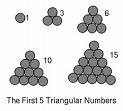First week of calculus, and the talk is all about limits and infinity... They say that one of Leibniz's earliest explorations into limits and infinite series was in finding the sum of the reciprocals of the triangular numbers, or$\sum_{1}^{\infty}\frac{2}{(n)(n+1)}\$The triangular numbers are the numbers that can be made by adding the counting integers 1, 1+2=3, 1+2+3=6, etc.. they also appear as the third number in on each row of Pascal's arithmetic triangle, so they are the combination of n things taken two at a time. The nth triangular number then, is$\dbinom{n}{2}$=$\frac{(n)(n+1)}{2}$The sequence of reciprocals would then$\frac{1}{1}+\frac{1}{3}+\frac{1}{6}+...+\frac{2}{(n)(n+1)}$But if we write them out using the$\frac{2}{(n)(n+1)}$=$\frac{2}{n}}$-$\frac{2}{n+1}$model for each term, then something interesting happens... writing 1 as$\frac{2}{1}-\frac{2}{2}$may seem silly at first, but if you follow that with 1/3 =$\frac{2}{2}-\frac{2}{3}\$ you realize that when you add these together, the alternating terms eliminate each other and the sum of the first two terms is just 2/1 - 2/3 or 1 1/3. Ok, the worlds slowest way to add one to one-third.. but now lets add the next few values 2/1-2/2 + 2/2-2/3+2/3-2/4+2/4-2/5 + 2/5 - 2/6 we see that all the underlined stuff disappears and we are left with the first term, 2, minus the last term, which is 2/(n+1) for whatever term number we are at.. so the sum of the first five terms, 1+1/3+1/6+1/10+1/15 = 2-2/6 or 1 2/3.

and if we wanted the first 20 terms we could just do 2 - 2/21 or 1 19/21. and if we added them up forever???? well what happens to 2/(n+1) as n gets bigger and bigger??? ....so the infinite sum will just approach a limit of two.

After I did this in class recently, I found this nice graphic demonstration at the wolfram demonstrations page;...a proof without words.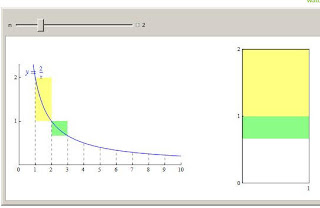"This problem was historically significant as it served as in inspiration for Leibniz to explore many more infinite series. Since he successfully solved this problem, he concluded that a sum could be found of almost any infinite series. (Boyer, 446-447)" ( Worcester Polytechnic Institute )

## Wednesday, 9 September 2009

### Roman Mobius Mosaics, A Myth??? Maybe

Still looking for some graphic of the supposed 3rd century Roman Mosaics that include a Mobius strip... and found another reference to them in :

Mathland: from flatland to hypersurfaces
By Michele Emmer[click on the graphics to enlarge]

Then I found this from :

Classical topology and combinatorial group theory
By John Stillwell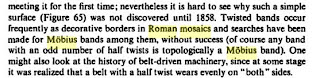So... somebody has it wrong....

## Tuesday, 8 September 2009

### Seeing Sounds, The Science of Cymatics

An interesting TED video for math and science classes, with some nice historical notes and some really neat visuals... I can See that sound in Two Notes... ok, showing my age...

## Monday, 7 September 2009

### Politically Correct Science, 1906 Style

Notice at the bottom of a page in the American Mathematical Monthly, Feb. 1906.

The Paris Academy of Sciences announces the Gutzman prize of 100,000 francs for communication with any star or planet other than mars. No affront to the Martians is intended [italics added]

Ok, this is in the period when Lowell was reporting and mapping "canals" on Mars from the 24 inch telescope at his observatory in Flagstaff. Were the French simply willing to accept that the Martians were too possible a target? I assume the prize was never paid..
--------------------------------------------------
Curiosity two.... reading Freeman Dyson's The 30 Greatest Problems of the Last 100 years. He commented that there were examples of Mobius strips in Roman Mosaics. I found a couple of other references, but not specific images. If anyone knows where I can find an image of such a mosaic, please advise..

## Saturday, 5 September 2009

### Pi and the 47 RoninIf you take the Asakusa Line from Shinagawa, just one stop away you will come to one of the most famous shrines in all of Tokyo, the Sengakuji Temple. It isn't the biggest, prettiest, or most ornate, but it is rich with the kind of history the Japanese love. This is the resting place of the 47 Ronin, one of Japan's most popular samurai stories.

"The story has all the elements for a Hollywood production: a good, noble guy who dies unfairly; a corrupt court official and cunning villain who is disliked by everyone but seems to be always ahead of the game; the good guy’s loyal subordinates who are totally determined to avenge their master’s death at whatever price, even with their own lives... In the end, the story has sparked the imagination and inspired the utmost respect from an entire nation for over 300 years."[Luis Estrada's Travel Blog]

I came across a mathematical reference to the story in a March 1908 article in the American Mathematical Monthly I received recently from Dave Renfro.

"In Tokyo, at the Buddhist temple of Sengakuji lie buried the forty-seven Ronin, the national heroes of feudal Japan. Just within the gate, in a two-storied building, swords, armor and other relics of these heroes are shown on payment of a fee. By the side of the path leading to the tombs is a well with the inscription, 'Here they washed it.' No one in Japan needs to be told that it was the bloody head they were bringing to the grave of their lord, that dead master for whom they considered it the highest privilege thus to forfeit all their lives. The popular reverence for these heroes is still attested not only by the incense perpetually kept burning before their tombs but in stranger fashion by the fresh visiting cards constantly left upon their graves. [To someone who is still there, do the Japanese still leave these visiting cards?]"

"All the world knows their exploit, but who knows that one of them, Shigekiyo Matsumura, was the greatest Asiatic mathematician of his age, who in his work
Sanso
, published in 1663, calculated the length of one side of a regular inscribed polygon of 32768 or 215 sides, obtaining 0.000095873798655313483 and thence for the value of pi 3.141592648, which is accurate to seven places of decimals, to eight significant figures..."

I would be thrilled if any of the folks who still read this in the Tokyo area would send a digital picture of the tomb of Matsumura so that I can add it to this note.

## Friday, 4 September 2009

### Counting Multisets, and A New Symbol for me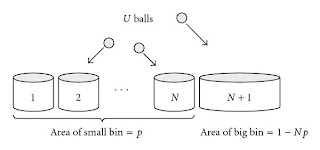Reading through The Endeavour, the blog of John D. Cook, I came across a new term and a symbol I had never seen, but actually quite like.

Here is a snip from what he wrote, "Suppose you have a class of 12 students. Each student will receive one of five letter grades: A, B, C, D, or F. At the end of the course, you tally up how many students received each grade. How many different ways could the tally turn out? For example, one possibility would be all A’s. Another would be three A’s, four B’s, four C’s, no D’s, and one F."

This is a special type of counting combinations, except that there can be more than one of each item. This is an example of what is called a multiset. Here is the explanation of "multiset" as it appears at Wikipedia,
"In mathematics, a multiset (or bag) is a generalization of a set. While each member of a set has only one membership, a member of a multiset can have more than one membership (meaning that there may be multiple instances of a member in a multiset, not that a single member instance may appear simultaneously in several multisets). The term "multiset" was coined by Nicolaas Govert de Bruijn in the 1970s. The use of multisets in mathematics predates the name "multiset" by nearly 90 years. Richard Dedekind used multisets in a paper published in 1888."

In fact another place on Wikipedia they mentioned that there are lots of other common names for multisets, and "Knuth also lists other names that were proposed for multisets, such as list, bunch, bag, heap, sample, weighted set, collection, and suite."

AHH, OK, I know "bag", I just never used is as a formal definition.
Ok, so how do you do a problem like that. In this case we have 5 identified things (the grades) and we want to select from this five, with replacement, until there are a total of 12. The classy way to do this uses a method called "stars and bars" and since some of my students read this, I will illustrate a little with a side note..

Suppose you are the waiter in a restaurant that sells three things, a single entree, a single type of beverage, and a dessert. You wait on a table of some number of people and each person orders one or more of the things.. Now being clever, you don't want to right down every order, so you divide your notepad into three columns by drawing two lines, remembering that the left space is for entrees, the middle one for drinks, and the last for dessert. At table two the four people ordered four entrees, two drinks and three desserts. You mark on your pad looks like xxxx | xx | xxx and when you get to the kitchen you can see what the order was..... x (stars) and | (bars) ...get it?

For 12 students and five grades we can imagine that we drew four vertical lines on a sheet of paper to separate it into five regions .... We could think of each region as representing a grade, A's to the left of the first line, B's between the first and second line, etc, with the Failing grades to the right of the fourth dividing line... the representation of three A’s, four B’s, four C’s, no D’s, and one F would then look like this; XXX | XXXX | XXXX | | X .

Note that if we identified the person who got each grade, we have created a very different problem, but for counting how many ways the 12 grades could be assigned without regard to who received each grade, we can look at the diagram and realize that if we re-order the 12 X's and four lines, we would get a different result... each order of lines and X's corresponds to exactly one assignment of 12 grades to the five letters. So the total number of ways of assigning the grades would be the same as the number of ways you could reorder the 16 characters, (lines and X's)..ie, how many ways can we pick 4 of them to be the lines???..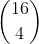So there are 1,820 possible grade tallies for 12 students and five grades.

Since you can stage the problem and use the notation that already exists for regular sets, it may not seem like a new symbol was necessary, but that leads, imho, to the student always trying to see the problem as a traditional combination rather than recognizing it is a different thing altogether. Perhaps that was his thinking when MIT professor Richard Stanley introduced the following symbol for the number of ways to select k things from a set of n with replacement;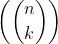From John Cook, (with additions) "There are several ways to think of what this symbol represents. The first is the number of ways to select k things from a set of n with replacement. (there are five boxes labeled A,B,C,D,F and we will put students into them with replacement)
A more concrete variation on the same idea is the number of ways to place k unlabelled balls (students) into n labeled urns (grades).

So we can writeIt looks a little strange to have the bottom value larger than the top, and I admit I have never seen it written that way, but then I haven't seen it used very much... but rest assured I WILL use it in probability problems this year. And how do you read it, well just extend the idea to include the new multiset term, I would read it as "N Multi-choose K".

## Tuesday, 1 September 2009

I was recently reading an interesting blog posted by Rick Regan at Exploring Binary
talking about the binary expansion of base ten numbers that are made up of repeating nines...

"I discovered a cool property of positive integers of the form 10n-1, that is, integers made up of n digits of 9s: they have binary representations that have exactly n digits of trailing 1s. For example, 9,999,999 in decimal is 100110001001011001111111 in binary. "

Rick went on to show that this must always be the case with some pretty simple algebra. He noticed that the part of the number preceding the repeating ones is the binary for 5n-1... ok here is a simple example... 102-1 =99 in base ten and in base two that is 1100011,.... the two repeating 1's at the end have a value of 22-1, the 11000 in front is the binary expression for 24 or 52-1.
Rick showed that 10n-1 can always be expressed as
(5n – 1) 2n + (2n – 1)...

It occurred to me as I read his blog, that we could also expres 10n-1 as

2n (5n) - 5n + 5n-1 by adding and subtracting 5n instead of 2n

Regrouping that as (2n-1 )5n +( 5n-1) shows that when expressed in base five, 10n -1 will begin with 2n-1 base five followed by a string of n fours,

9  = 1 4

99= 344

999= 12444

9999=1114444

A similar event happens (I think, but do not prove) for any (pq)n -1 if p and q are (relatively?) Prime when expressed in base p or base q
... for example instead of 10n-1, if we used 6n-1 we would get
2n(3n) - 2n+2n-1 In base two that would show up as the base two number for 3n followed by a string of n ones.

For example 63-1 in base two is 11010111. The front part, 11010, is 26 or 33-1 in base ten, and then followed by three ones..

We could do the same switch to express it in base three.
The same number (63-1) in base three would give 21222, with 21 in front being the base three for 7 or 23-1 and then followed by a string of three twos..

If you want to explore binary problems, he Rick also has a convertor on his web page to go between decimal and binary. Of course you can always use Wolfram-alpha [I have a little "alpha" gadget on my I-google page to keep it handy]... By writing "convert 315 base 8 to base 3" it gave me 211213.

As a side note, I find that using alternate bases helps lots of kids really get a feel for polynomials they never had before (and perhaps a deeper understanding of the decimal system they thought they had fully mastered. Quickly students, can you see why 11010111 in base two would be 3113 in base four?